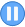﻿

## GMAT Practice Question Set #19 (Question 55-57)

Problem Solving Question #56: Function Constraints
 Time 0 0 : 0 0 : 0 0Among the functions given below, which one satisfies the equation f(x + y) = f(x) + f(y) for all positive numbers x and y? (A)f(x) = -2x (B)f(x) = 2/x (C)f(x) = sqrt(x) (D)f(x) = x + 1 (E)f(x) = x^2GMATTM is a registered trademark of the Graduate Management Admission CouncilTM. The Graduate Management Admission CouncilTM does not endorse, nor is it affiliated in any way with the owner or any content of this web site.